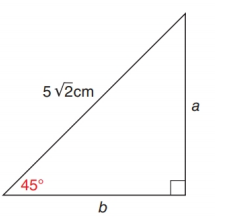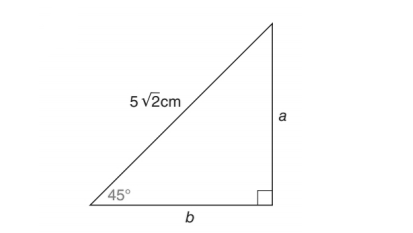Chapter 11.2, Problem 19E### Elementary Geometry for College St...

6th Edition
Daniel C. Alexander + 1 other
ISBN: 9781285195698

#### Solutions

Chapter
Section### Elementary Geometry for College St...

6th Edition
Daniel C. Alexander + 1 other
ISBN: 9781285195698
Textbook Problem
1 views

# In Exercise 17 to 22, use either the sine ratio or the cosine ratio to find the length of the indicated sides of the triangle correct to the nearest tenth of a unit.To determine

To find:

The length of the indicated sides of the following triangle,Explanation

Consider the following figure,

General formula for cosine ratio and sin ratio is given below,

From the given figure the value 52cm is the hypotenuse value since it is opposite to right angle.

Then b. is the length of the leg adjacent to 45 and a is the length of leg opposite to 45.

Therefore,

cos45=b52b=52×cos45

Using a scientific calculator in degree mode, follow the following key sequence,

45cos0

### Still sussing out bartleby?

Check out a sample textbook solution.

See a sample solution

#### The Solution to Your Study Problems

Bartleby provides explanations to thousands of textbook problems written by our experts, many with advanced degrees!

Get Started

#### Find the value of the sum. 32. i=1n(3+2i)2

Single Variable Calculus: Early Transcendentals, Volume I

#### In Problems 25-34, use the matrices below. Perform the indicated operations.

Mathematical Applications for the Management, Life, and Social Sciences

#### Finding a Limit In Exercises 47-62, find the limit. limx4x+53x4

Calculus: Early Transcendental Functions (MindTap Course List)

#### True or False: f(a)=lima0f(a+h)f(a)h.

Study Guide for Stewart's Single Variable Calculus: Early Transcendentals, 8th